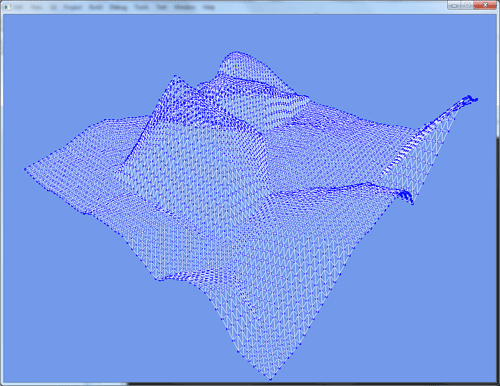## Terrain Mesh Simplification

### For: CSC 572, Graduate Computer Graphics with Zoe Wood

#### Download: Source or Win32 BinaryThe terrain mesh at full resolution, 8192 triangles.The simplified terrain mesh, ~200 triangles.

For my final project I wanted to implement a mesh simplification algorithm for terrain meshes. I decided to try a technique that takes advantage of the features of a heightmap in order to do quick error calculations that are comparable in accuracy to Progressive Meshes, but in a much shorter amount of time.

The heightmap is generated using a linear combination of a traditional Fractal Landscape technique and Veroni Diagrams. The Fractal Landscape provides a good amount of noise while the Veroni Diagrams produce large, distinct features in the terrain. Explanation of this technique of terrain generation can be found in the beginning section of Realtime Procedural Terrain Generation.

Like in other mesh simplification algorithms, an error for collapsing each edge is calculated and stored in a priority queue. The least cost edge collapse is pulled off the queue, applied, then the appropriate neighboring edges are recalculated and reinserted into the queue. Edge collapses are applied until the desired error or face count has been achieved.

In other techniques, an optimal position for the resulting vertex from an edge collapse is calculated by minimizing an error function. In this algorithm, we simply chose one of the original vertices that generates the least error. Some edge collapses can cause undesirable geometry. For example, if an edge collapse will produce a triangle that has no area, it is invalid and thrown out. We also prevent faces from becoming vertical or pointing upside down. The normals for each face must have some positive Y component. More checks are enforced to prevent the square shape of the heightmap from being degraded.

Since our original mesh has no overlapping triangles in the Y-axis, and we enforce that all new triangles must "point up", we know that any resulting simplified mesh must also not have any overlapping triangles. We can use this knowledge to associate any point on the original heightmap with one of the triangles of the simplified mesh. Using a triangle rasterization algorithm, we can easily figure out which points on the original heightmap we want to use in the error calculation.

In practice, this did not work very well by itself. Very long, skinny triangles were produced that spanned nearly the entire heightmap, but did not actually cover any of the vertices. The rasterization function was modified so that heightmap points that were close, but not neccesarily being overlapped are used in the error calculation as well. This means that heightmap points are used multiple times among different triangles.

To calculate the error, we calculate the plane of the triangle, then use a simple point-plane distance calculation between the plane and each corresponding heightmap point. The error metric for an edge collapse becomes the sum of the squared distances for all triangles that will be modified by that edge collapse.

Future work on this project would include a comparison with other mesh simplification techniques such as ROAM and Quadric Error Metrics.